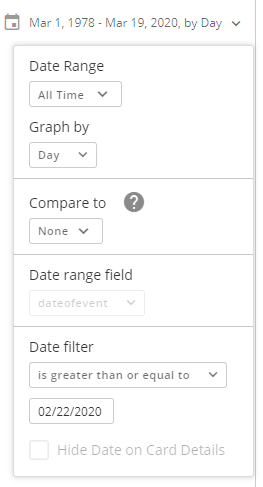# How to: Use a custom date range that rolls everyday

Hey everyone,

I am currently stuck at a point where I need to find the difference between current date and the feb 22nd to plit my results. But the current date needs to be plotted each day from feb 22nd.

I am using this for each day (Singel Value Chart) and it works, however I can't use this to plot a line graph as it only takes in the current day. I need to move this to a rolling date difference from the 22nd Feb.

DATEDIFF(CURRENT_DATE(), '2020-02-22')

Any suggestions?

• Are you trying to show on your x-axis the days since Feb 22nd so that it would display 1, 2, 3, 4, 5, etc...? If so, you would need the dates for each of those days in your dataset and then you could do a datediff between that date and Feb 22nd. What columns do you have in your dataset?

Also, if you do have the dates in your dataset, you could use the Date Range Filter and choose Graph By Day and also choose Greater Than Feb 22nd. This will put the date of each day on your x-axis.Hope this helps.

• Hey Mark, thanks for the reply.

Here is what I am trying to achieve:
I have a beastmode calculatation that gives me the sum of the loss from Feb 22nd till Current Date in a Single Value Chart. I then divide that by the number of the days to give me an average per day.

So for example, loss on:
Feb 22nd - \$1000

Feb 23rd - \$1800

Feb 24th - \$2000

Feb 25th - \$1200

Then the beastmode would spit out: \$1500 per day. Now I want to make a plot that would essentially spit this value every day from Feb 22nd...

So imagine a line chart that would plot the folllowing:

Feb 22nd - \$1000

Feb 23rd - \$1400 (Average of previous and current day)

Feb 24th - \$1600 (Average of all days between 22nd Feb and Current)

Feb 25th - \$1500 (Average of all days between 22nd Feb and Current)

and so forth... hope this made my initial question clear?

• Thank you so much, that makes sense!

• @jaeW_at_Onyx this was by far the best explained tutorial. Thank you!